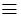## Indicators

•Cited by SciELO
•Access statistics

•Similars in SciELO

## Print version ISSN 1405-5546

#### Abstract

MAITRA, Subhamoy; SUBBA RAO, Y. V.; STANICA, Pantelimon  and  GANGOPADHYAY, Sugata. Nontrivial Solutions to the Cubic Sieve Congruence Problem: x3y2z mod p. Comp. y Sist. [online]. 2009, vol.12, n.3, pp.253-266. ISSN 1405-5546.

In this paper we discuss the problem of finding nontrivial solutions to the Cubic Sieve Congruence problem, that is, solutions of x3 = y2z (mod p), where x,y,z <and x3 y2z. The solutions to this problem are useful in solving the Discrete Log Problem or factorization by index calculus method. Apart from the cryptographic interest, this problem is motivating by itself from a number theoretic point of view. Though we could not solve the problem completely, we could identify certain sub classes of primes where the problem can be solved in time polynomial in log p. Further we could extend the idea of Reyneri's sieve and identify some cases in it where the problem can even be solved in constant time. Designers of cryptosystems should avoid all primes contained in our detected cases.

Keywords : Cubic Sieve Congruence; Discrete Log Problem; Prime Numbers.

· abstract in Spanish     · text in English     · English (pdf )All the contents of this journal, except where otherwise noted, is licensed under a Creative Commons Attribution License